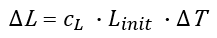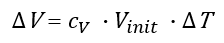# Thermal Expansion Calculator

Easily calculate the thermal expansion in length, volume or the change in density with a change in temperature. This thermal expansion calculator can be used to calculate linear or volumetric expansion of a material. Supports a variety of input metrics such as Celsius, Fahrenheit, Kelvin, length and volume in both metric and imperial units.

Share calculator:

Embed this tool:
get code

## Using the Thermal Expansion calculator

To understand how to make good use of this online thermal expansion calculator, we need to begin by explaining what thermal expansion is. To begin - let us examine what temperature actually is.

Temperature is a monotonic function of the average molecular kinetic energy of a substance so when a material is heated the sum of the kinetic energy of its molecules increases, and vice versa. If the substance is unconstrained and its temperature is increased its volume expands in all dimensions (thermal expansion) and its density decreases. Simply put, most solid materials expand upon heating and contract when cooled. There are also exceptions: some materials which contract when their temperature increases, but these effects are quite limited and only occur in specific temperature ranges.

The relative expansion (strain) of a material divided by the change in temperature is what is known as its coefficient of thermal expansion (CTE). Usually you would know the coefficient of linear expansion (CLTE) of a material and its initial and end temperature, which will allow you to calculate the linear expansion across any of its dimensions, its volumetric expansion, as well the change in density.

This calculator is an easy to use versatile tool you can use as a:

• linear thermal expansion calculator
• volumetric thermal expansion calculator
• density change under temperature change calculator

Simply enter the CLTE (see reference table below), the initial and final temperature and the initial length, volume or density to calculate the final length, volume or density. It supports both imperial and metric units for volume and pressure and 5 different temperature scales: Kelvin, Celsius, Fahrenheit, Rankine and Reamur.

The units for volume supported by the tool are: mm3, cm3, m3, ml, L (litre), gallons, fluid ounces, cubic inches, cubic feet and cubic yards. Units for density supported by the tool are g/m3, kg/m3, oz per cu in, lb per cu ft, grams per litre, and others.

## Thermal Expansion formulas

This thermal expansion calculator uses the following formula for linear thermal expansion of a particular dimension of a solid object:where ΔL is the change in length, cL is the coefficient of linear thermal expansion of the material (CLTE) in K-1, Linit is the initial length and ΔT is the change in temperature in Kelvins. To find the final length simply add the change to the initial length.

The formula for volumetric thermal expansion of a solid object used in the calculator is:where ΔV is the change in volume, cV is the coefficient of volumetric thermal expansion of the material in K-1 (3 times the coefficient of linear thermal expansion), Vinit is the initial volume and ΔT is the change in temperature in Kelvins. To find the final volume simply add the change to the initial volume.

For change in density we subtract the starting density divided by (1 + cV · ΔT) from the starting density.

## Coefficient of thermal expansion of common materials

The coefficient of thermal expansion describes how the size of an object changes with a change in temperature: it is calculated as the fractional change in size per degree change in temperature at constant pressure. This table presents linear coefficients, but aerial and volumetric ones can easily be extracted by multiplying the linear ones by 2 or 3, respectively. As a general rule, high melting point materials are more likely to have lower thermal expansion. The values in the following table are derived from reference 1 and can be useful in using the calculator.

Linear coefficient of thermal expansion for different metals
MaterialLinear CTE (α) from 18°C to 100°C (212°F) in ppm/K (10-6/K)
Pure Silicon (Si) 2.6 - 3.3
Pure Tungsten (W) 4.5 - 4.6
Pure Molybdenum (Mo) 4.8 - 5.1
Pure Chromium 4.9 - 8.2
Pure Titanium 8.4 - 8.6
High-chromium gray cast iron 9.3 - 9.6
Maraging steel 8.6 - 11
Ferritic stainless steel 9.3 - 12
Martenistic stainless steel 9.5 - 12
High-carbon high-chromium cold work tool steel 10 - 12
Wrought iron 11
Shock-resisting tool steel 11 - 12
Structural steel 12
Resulfurized carbon steel 11 - 14
Chromium alloy steel 9.4 - 15
High-strength low-alloy steel (HSLA) 12 - 13
High strength structural steel 9.9 - 13
Pure Iron 12 - 13
Nickel copper 11 - 16
Tungsten alloys 14
Silver alloys 18 - 20
Manganese (Mn) 22
1xx.x series commercially pure cast aluminum 18 - 24
3xx.x series cast aluminum silicon+copper or magnesium 16 - 23
Pure Calcium (Ca) 22
8xx.x series cast aluminum tin 23
Zinc aluminum 22 - 28
Pure Magnesium (Mg) 25 - 26
Zinc copper 33 - 35
Pure Sodium (Na) 69 - 71
Pure Phosphorus (P) 125

For a more complete list see the reference or, if available, use the manufacturer-provided coefficient.

Here are the CLTEs of some other materials, at 20°C which might also be relevant in certain uses of this tool:

Linear coefficient of thermal expansion for different non-metal materials
MaterialLinear CTE (α) at 20°C (68°F) in ppm/K (10-6/K)
Quartz (pure glass) 0.59
Diamond 1
Glass 8.5
Concrete 12
Ice 51
PVC 52
Oak 54
Water 69